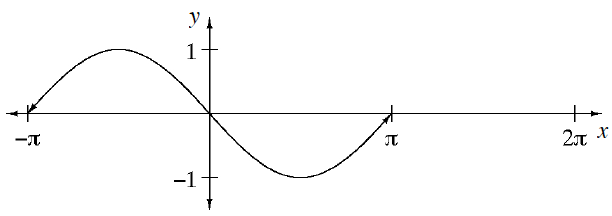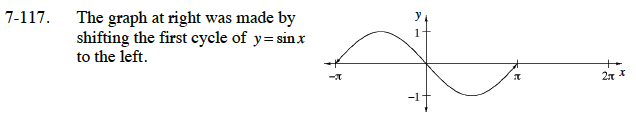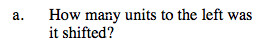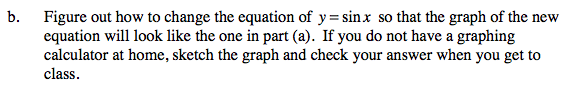Home > CCA2 > Chapter 7 > Lesson 7.2.1 > Problem7-117

7-117.
1.The graph at right was made by shifting the first cycle of y = sin x to the left. Homework Help ✎

1. How many units to the left was it shifted?

2. Figure out how to change the equation of y = sin x so that the graph of the new equation will look like the one in part (a). If you do not have a graphing calculator at home, sketch the graph and check your answer when you get to class.It is shifted π units to the left.In the general equation y = sin(xh) + k, which parameter moves the graph horizontally?